Processing ......FreeComputerBooks.com Links to Free Computer, Mathematics, Technical Books all over the World

Introduction to Mathematical Philosophy
Top Free C++ Books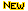- 100% Free or Open Source!
• Title Introduction to Mathematical Philosophy
• Author(s) Bertrand Russell
• Publisher: George Allen and Unwin, UK (1919); Martino Fine Books (2017 Reprint); eBook (Public Domain)
• License(s): Public Domain Certification, CC BY-SA 3.0 US
• Hardcover/Paperback 220 pages
• eBook HTML, PDF, ePub, Kindle (Mobi), TeX, etc.
• Language: English
• ISBN-10/ASIN: 1684221447
• ISBN-13: 978-1684221448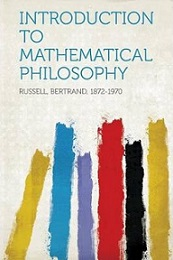Book Description

Introduction to Mathematical Philosophy has been a seminal work for more than nine decades. It gives the general background necessary for any serious discussion on the foundational crisis of mathematics in the beginning of the twentieth century.

Requiring neither prior knowledge of mathematics nor aptitude for mathematical symbolism, the book serves as essential reading for anyone interested in the intersection of mathematics and logic and in the development of analytic philosophy in the twentieth century. Russell offers to his readers a penetrating discussion on certain issues of mathematical logic that embodies the dawn of modern analytic philosophy.

• Bertrand Russell was a British philosopher, logician, mathematician, historian, writer, social critic, political activist, and Nobel laureate.
Reviews, Rating, and Recommendations: Related Book Categories: Read and Download Links:Similar Books:
•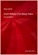Gödel Without (Too Many) Tears (Peter Smith)

How is this remarkable result of Gödel's Theorems established? This short book explains. The aim is to make the Theorems available, clearly and accessibly, even to those with a quite limited formal background.

•Introduction to Mathematical Logic (Vilnis Detlovs, et al)

This book explores the principal topics of mathematical logic. It covers propositional logic, first-order logic, first-order number theory, axiomatic set theory, and the theory of computability. Discusses the major results of Gödel, Church, Kleene, Rosser, and Turing.

•A Friendly Introduction to Mathematical Logic (Chris Leary)

In this user-friendly book, readers with no previous study in the field are introduced to the basics of model theory, proof theory, and computability theory, leading to rigorous proofs of Gödel's First and Second Incompleteness Theorems.

•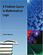A Problem Course in Mathematical Logic (Stefan Bilaniuk)

It is intended to serve as the text for an introduction to mathematical logic for undergraduates with some mathematical sophistication. It supplies definitions, statements of results, and problems, along with some explanations, examples, and hints.

•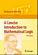A Concise Introduction to Mathematical Logic (W. Rautenberg)

This is a well-written introduction to the beautiful and coherent subject of mathematical logic. It contains classical material such as logical calculi, beginnings of model theory, and Goedel's incompleteness theorems, as well as some topics motivated by applications.

•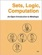Sets, Logic, Computation: An Open Introduction to Metalogic

It covers naive set theory, first-order logic, sequent calculus and natural deduction, the completeness, compactness, and Löwenheim-Skolem theorems, Turing machines, and the undecidability of the halting problem and of first-order logic.

Book Categories
 :All CategoriesTop BooksRecent BooksMiscellaneous BooksComputer LanguagesComputer ScienceData Science/DatabasesElectrical EngineeringJava and Java EE (J2EE)Linux and UnixMathematicsMicrosoft and .NETMobile ComputingNetworking and CommunicationsSoftware EngineeringSpecial TopicsWeb Programming
Other Categories